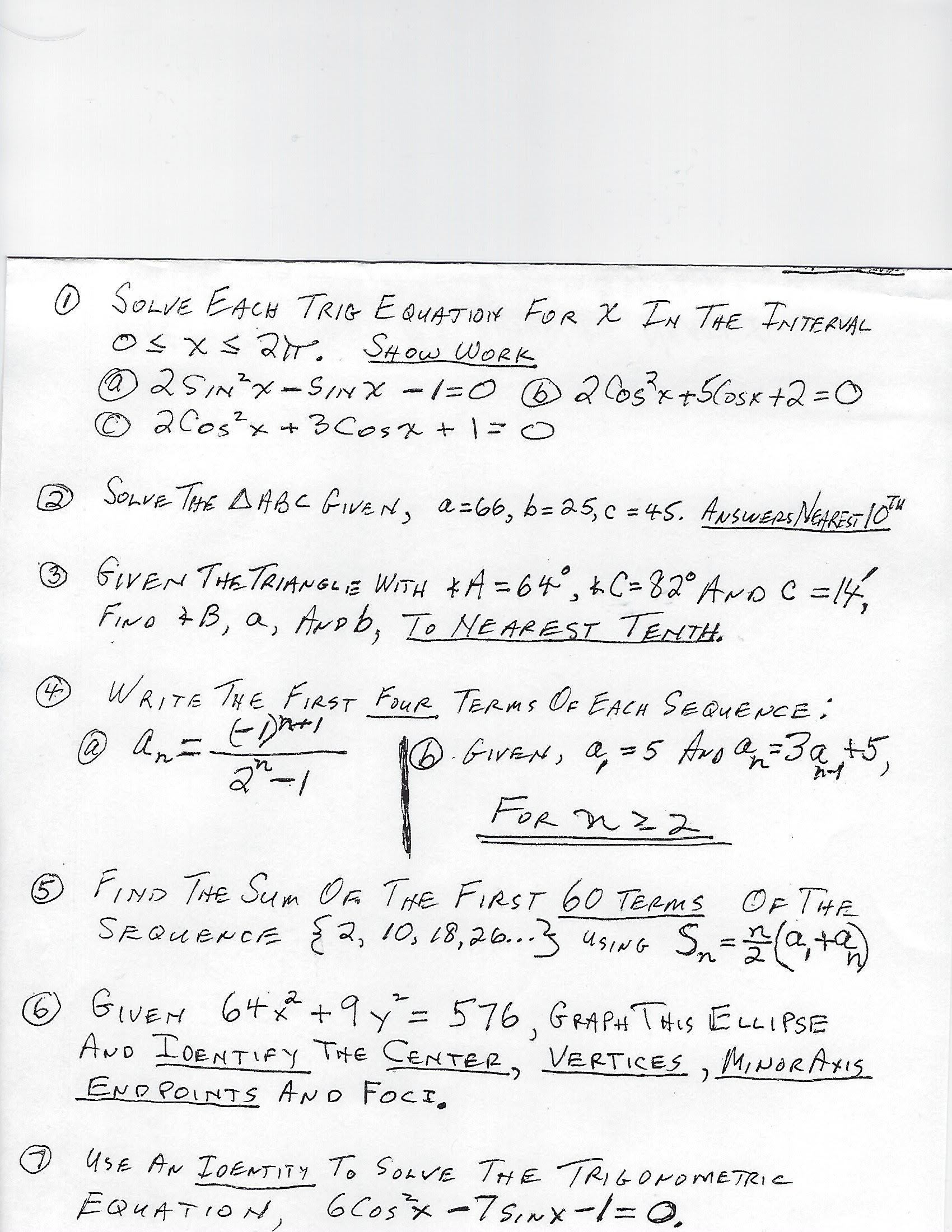# O SOLVE EACH TRIG E QUATION FOR X IN THE TNTERUALOs xs 2r2SINX-SINX -1=0 B2o5x+5CosK +2=D0©2Cos?x + 3Cosx + | =0SHOW WORKSonve THE AHBC GiveN,a-66, b= 25,c = 45. ANSWEPS NERES 10"(2)%3DGIVEN THETRIANGLE WITH *A = 64°, kC=82° AroC =14,FINo 7B, a, Arob, To Ne AREST TENTH.%3DWRITE THE FIRST FOUR TERms Oe EACH SEQUENCE:(4)An-6.GiVEN, a, =5 Avo a=3a +5.la)FOR nz 2FIND THE Sum Oa THE FIRST 60 TERMSOF THESn-G+)SRQUENCE {2, 10, L8,26..3 usiG Sn=,+USINGGIVEM 64* +9y= 576, GRA PAt THIS ELLIPSEAND IDENTIFY THE CENTER, VERTICES ,MINORAXISEND POINTS AND FOCI,9.O UsE AN IDENTITY To SoavE THE TRIGONOMETRIC6 Cosx -7sinx-1=0.EQUATION,

Question
6 views

help me with the solution and explanation of the question 4help_outlineImage TranscriptioncloseO SOLVE EACH TRIG E QUATION FOR X IN THE TNTERUAL Os xs 2r 2SINX-SINX -1=0 B2o5x+5CosK +2=D0 ©2Cos?x + 3Cosx + | =0 SHOW WORK Sonve THE AHBC GiveN, a-66, b= 25,c = 45. ANSWEPS NERES 10" (2) %3D GIVEN THETRIANGLE WITH *A = 64°, kC=82° AroC =14, FINo 7B, a, Arob, To Ne AREST TENTH. %3D WRITE THE FIRST FOUR TERms Oe EACH SEQUENCE: (4) An- 6.GiVEN, a, =5 Avo a=3a +5. la) FOR nz 2 FIND THE Sum Oa THE FIRST 60 TERMS OF THE Sn-G+) SRQUENCE {2, 10, L8,26..3 usiG Sn=,+ USING GIVEM 64* +9y= 576, GRA PAt THIS ELLIPSE AND IDENTIFY THE CENTER, VERTICES ,MINORAXIS END POINTS AND FOCI, 9. O UsE AN IDENTITY To SoavE THE TRIGONOMETRIC 6 Cosx -7sinx-1=0. EQUATION, fullscreen
check_circle

Step 1

a) For first four terms we take n=1,2,3,4

Plug n=1 first.

First term = 1

Step 2

Plug n=2 then.

Second term = -1/3

Step 3

Plug n=3 then.

Third &nb...

### Want to see the full answer?

See Solution

#### Want to see this answer and more?

Solutions are written by subject experts who are available 24/7. Questions are typically answered within 1 hour.*

See Solution
*Response times may vary by subject and question.
Tagged in
MathCalculus

### Other